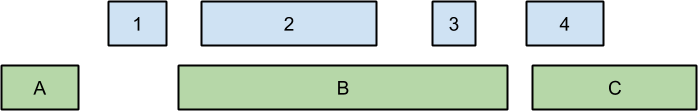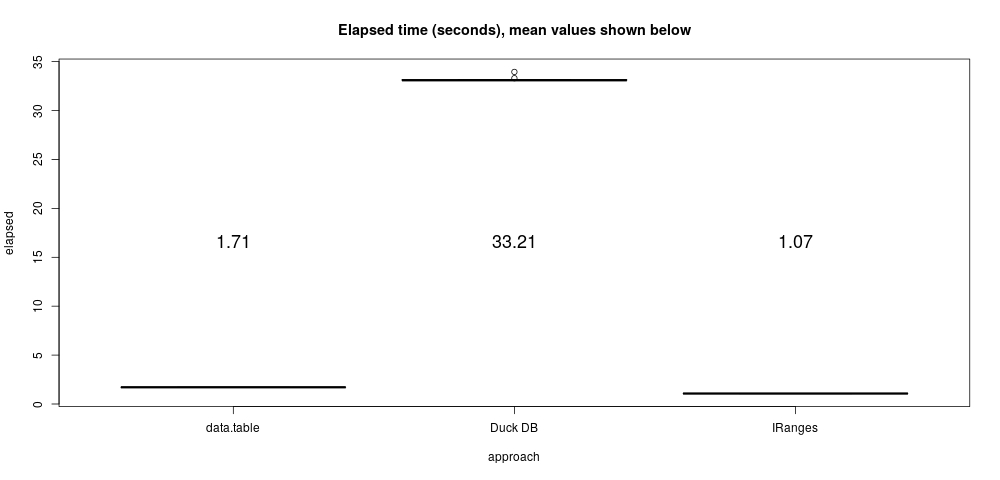# Overlap joins on genomic data

Finding overlapping regions between two sets of coordinate values is a common problem in computational genomics and other disciplines. At least two R packages can very rapidly perform this computation: the IRanges package by Pages, Aboyoun and Lawrence in the bioconductor1 and the widely popular data.table package by Dowle, Short, Lianoglou, Srinivasan, and others2.

## The Overlap Join

The overlap join finds overlapping regions between two tables of coordinates. The following figure, adapted from Alex Poliakov at Paradigm4, illustrates a simple example. The right to left extent of the blue and green boxes depicts two sets of ranges, respectively.Two sets of ranges

The overlap join operation finds ranges with overlapping extents, for example the green box labeled “B” overlaps with the blue boxes “2” and “3”, and the green box labeled “C” overlaps with the blue box labeled “4.” In practice, overlap joins may be performed on tables with millions of ranges.

The example presented below finds overlaps between genomic variant coordinates from the 1000 genomes project3, and a list of human gene coordinates from https://www.pharmgkb.org/ 4. The example proceeds as follows:

1. Find variant and gene overlaps
2. Count the number of variants that overlap each gene by gene symbol
3. Order the genes by number of variant overlaps

By genomic standards, this is a small problem. The raw data files consume about 1.9 GB compressed, but the actual range data are pretty small: about 9 million variant coordinates and only about 2,100 gene ranges. As you can imagine, a lot of the work in this problem involves parsing the raw data to uncover those ranges.

download.file("ftp://ftp-trace.ncbi.nih.gov/1000genomes/ftp/release/20130502/ALL.chr7.phase3_shapeit2_mvncall_integrated_v5a.20130502.genotypes.vcf.gz", destfile = "ALL.chr7.phase3_shapeit2_mvncall_integrated_v5a.20130502.genotypes.vcf.gz")
download.file("https://github.com/bwlewis/duckdb_and_r/blob/master/genes.tsv?raw=true", destfile = "genes.tsv")

## Data setup, or, pipelined parallelism is the best parallelism!

The following code parses the input variant files into R data frames with position, reference allele, and alternate allele columns. It results in a list called variants with two data frames of variant coordinates and values, one for chromosome 7 and one for chromosome 8.

library(parallel)
library(data.table)

f <- function(file)
{
cmd <- sprintf("zcat %s | sed '/^#/d;/IMPRECISE/d' |  cut -f 2,4,5", file)
name <- gsub(".phase.*","", file)
print(name)
x <- read.table(pipe(cmd), stringsAsFactors = FALSE)
names(x) <- c("start", "ref", "alt")

# Process extra comma-separated alleles
idx <- grepl(",", x[["alt"]])
s <- strsplit(x[idx, "alt"], ",")
# replace these rows with 1st alternate allele
x[idx, "alt"] <- vapply(s, function(z) z, "")
# Add new rows corresponding to other listed alternates
ref <- x[idx, 1:2]
N   <- vapply(s, length, 1) # numbers of alternates by row
alt <- Map(function(i)
{
j <- which(N == i)
cbind(ref[j, ], alt = vapply(s[j], function(z) z[i], ""))
}, seq(2, max(N)))
rbindlist(c(list(x), alt))
}
files <- dir(pattern = "*.vcf.gz")
variants <- mcMap(f, files)
# name data frames by chromosome number:
names(variants) <- gsub("^ALL.chr", "", gsub(".phase.*", "", names(variants)))

This step takes about 160 seconds on my laptop (to parse 9,362,139 variants on both chromosomes 7 and 8).

The parsing program is complicated by the doubly-delimited data file format. In particular more than one alternate allele may be listed per line separated by commas (the remainder of the file is tab separated). R handles the extra format complexity with relative ease and efficiency. Parsing multiple alternate alleles adds only a dozen lines to the R program and runs very fast. Languages like R, Python, Perl, awk, etc. excel at handling complex data formats like this.

I use shell utilities here to help uncompress and process the TSV files. R’s pipe connection object makes it easy to operate on pipelines of shell commands conveniently from R. (The data.table package includes similar functionality but, somewhat unfortunately, using a different syntax).

Because the above processing pipeline includes several stages (zcat, sed, cut, read.table), there is a fair amount of parallel computation going on. The script additionally uses the explicitly parallel mcMap function which may lead to some over-committing of CPU resources, but still generally improves performance.

Note that the script omits imprecisely specified variants, further described here: http://goo.gl/PFsIsP. Handling imprecise variants would add a lot of complexity to this simple example.

The genes.tsv file is small and fast to parse by comparison. The following script loads the listed gene ranges corresponding to chromosomes 7 and 8 into a data frame. Note that some listed genes contain incomplete information (NA) and are removed and that we’re using the GRCh37 reference genome positions.

cmd <- "cat genes.tsv | cut -f 6,13,14,15 | grep 'chr[7,8]' | sed -e 's/chr7/7/;s/chr8/8/'"
p <- pipe( cmd, open="r")
genes <- na.omit(read.table(p, stringsAsFactors = FALSE, header = FALSE, sep = "\t"))
close(p)
names(genes) <- c("gene", "chromosome", "start", "end")
genes <- genes[genes[["start"]] > 0 & genes[["end"]] > 0, ]

## R data.table

The popular R data.table package includes a fast overlap join function called foverlaps. The following code uses that function to compute the overlap join between variants and genes for each chromosome, and then ranks genes by the by the count of overlapping variants per gene. The code runs in parallel over each chromosome using the mcMap function.

overlap <- function(chromosome)
{
x <- variants[[as.character(chromosome)]]
x[["end"]] <- x[["start"]] + nchar(x[["ref"]])

g <- genes[genes[["chromosome"]] == chromosome, c(1, 3, 4)]
setDT(g, key = c("start", "end"))
setDT(x)
foverlaps(x, g, nomatch = 0)[, .N, by = gene]
}
t1 <- replicate(10, system.time(ans.DT <<- rbindlist(mcMap(overlap, c(7, 8)))))
ans.DT <- ans.DT[order(ans.DT[["N"]], decreasing = TRUE), ]

Example output of this step looks like:

head(ans.DT, n = 10)
#  CSMD1 CNTNAP2    SGCZ    SDK1   MAGI2  PTPRN2  DLGAP2   CSMD3    NRG1   AUTS2
# 147459   76073   52174   45067   43984   41662   40362   34397   33001   31275 

## R IRanges

We can similarly compute counts of overlapping variants and genes using the IRanges package from the bioconductor.

If you don’t already have it, you can install the IRanges package with:

source("http://bioconductor.org/biocLite.R")
biocLite("IRanges")

This example is almost identical to the data.table version above:

library(IRanges)
overlap = function(chromosome)
{
x <- variants[[as.character(chromosome)]]
ir1 <- IRanges(start=x[["start"]], end=x[["start"]] + nchar(x[["ref"]]))
g <- genes[genes[["chromosome"]] == chromosome, c(1, 3, 4)]
ir2 <- IRanges(start = g[["start"]], end = g[["end"]])
ans <- findOverlaps(ir1, ir2)
data.frame(gene = g[["gene"]], count = countRnodeHits(ans))
}
t2 <- replicate(10, system.time(ans.IR <<- rbindlist(mcMap(overlap, c(7, 8)))))
ans.IR <- ans.IR[order(ans.IR[["count"]], decreasing = TRUE), ]

The IRanges counts match those from the data.table example, with the exception that IRanges also includes zero count results.

## Duck DB

Let’s try DuckDB on this problem. I’m not a SQL expert, but the query I wrote below is a pretty intuitive implementation of the overlap join and actually is quite readable. But I wonder if that query can be improved from a performance standpoint? (On the other hand, if it can, shouldn’t the query optimizer figure that out?)

Note that the example below combines the variants data frames into a single ‘variants’ table in DuckDB, adding a ‘chromosome’ number column to identify each sub-table. This makes for a single, simpler query.

library(duckdb)

variants[][["chromosome"]] <- 7
variants[][["chromosome"]] <- 8
variants <- rbindlist(variants)
duckdb_register(con, "genes", genes)
duckdb_register(con, "variants", variants)

Q <- 'SELECT G.gene, COUNT(V.ref) AS "count"
FROM genes G JOIN variants V
ON G.chromosome = V.chromosome
AND (G.start <= (V.start + length(ref)) AND G.end >= V.start)
GROUP BY 1 ORDER BY 2 DESC'
t3 <- replicate(10, system.time({ans.DB <<- dbGetQuery(con, Q)}))

The output counts are the same as those produced by IRanges and data.table.

The data.table package is usually the fastest thing going, but in this example it’s bested slightly by the Bioconductor IRanges package in performance. However, note that data.table’s foverlaps function has a lot of additional capabilities not used here (see the documentation).

The SQL version is nicely readable and understandable in this example, but for reasons unknown to me the performance is quite slow. Perhaps a better SQL query can be written?

timings <- rbind(data.frame(approach = "data.table", elapsed = t1[3, ]),
data.frame(approach = "IRanges", elapsed = t2[3, ]),
data.frame(approach = "Duck DB", elapsed = t3[3, ]))
boxplot(elapsed ~ approach, data = timings, main = "Elapsed time (seconds), mean values shown below")
m <- aggregate(list(mean = timings[["elapsed"]]), by = list(timings[["approach"]]), FUN=mean)
text(seq(NROW(m)), y = max(m[["mean"]])/2, labels = round(m[["mean"]], 2), cex = 1.5)1. Gentleman R.C., Carey V.J., Bates D.M., Bolstad B., Dettling M., Dudoit S., Ellis B., Gautier L., Ge Y., Gentry J., Hornik K., Hothorn T., Huber W., Iacus S., Irizarry R., Leisch F., Li C., Maechler M., Rossini A.J., Sawitzki ., Smith C., Smyth G., Tierney L., Yang J.Y. and Zhang J. (2004) Bioconductor: open software development for computational biology and bioinformatics. Genome Biol. 5(10): R80.

2. An integrated map of genetic variation from 1,092 human genomes, McVean et Al, Nature 491, 56-65 (01 November 2012) doi:10.1038/nature11632.

3. M. Whirl-Carrillo, E.M. McDonagh, J. M. Hebert, L. Gong, K. Sangkuhl, C.F. Thorn, R.B. Altman and T.E. Klein. “Pharmacogenomics Knowledge for Personalized Medicine” Clinical Pharmacology & Therapeutics (2012) 92(4): 414-417.